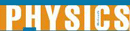# Work and Potential Energy - Research Article from World of Physics

This encyclopedia article consists of approximately 5 pages of information about Work and Potential Energy.
 This section contains 1,410 words (approx. 5 pages at 300 words per page) View a FREE sampleWork is performed whenever a force moves an object. When a person lifts an object, he or she is performing work. More work is done if the object is heavier or if it is lifted higher. Work, then, depends on the force acting on the object as well as the distance it is moved. The amount of work done (W) can be calculated by multiplying the magnitude of the force acting on an object (F) by the distance the object moves (d), using the equation W = F. d. Force and distance are vector quantities, that is, they have both a magnitude and a direction. The direction of the force and distance are important in work calculations, as will be discussed below. Using the English system of measurement, the force is measured in pounds (lb) and the distance is measured in feet (ft...

(read more)

 This section contains 1,410 words (approx. 5 pages at 300 words per page) View a FREE sampleMore summaries and resources for teaching or studying Work and Potential Energy.
CopyrightsWork and Potential Energy from Gale. ©2005-2006 Thomson Gale, a part of the Thomson Corporation. All rights reserved.